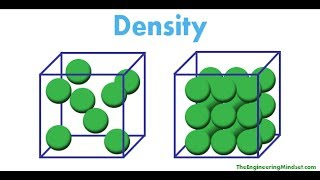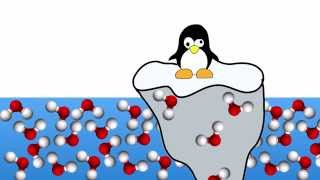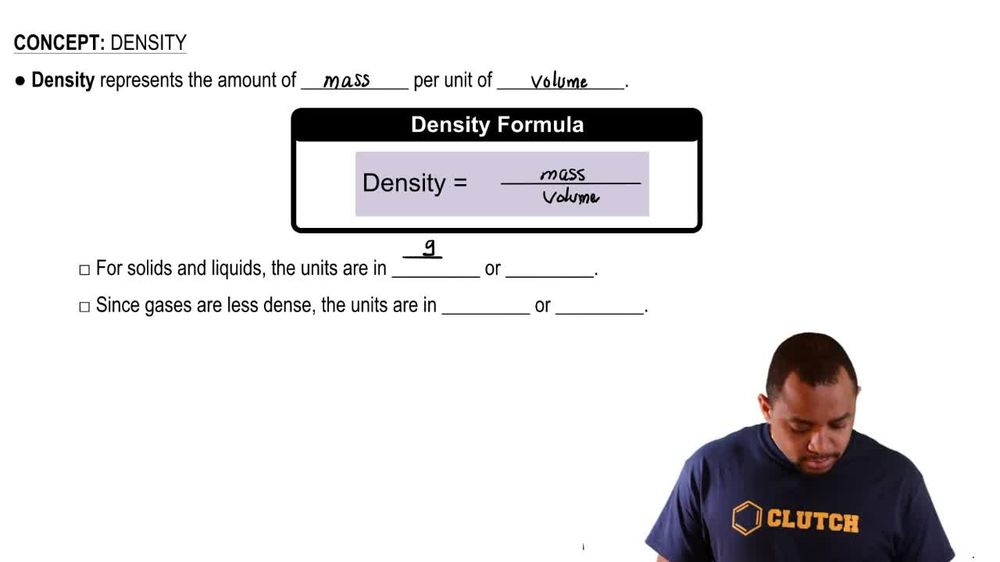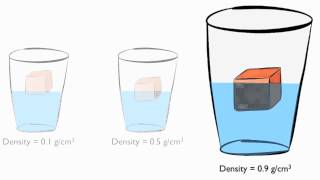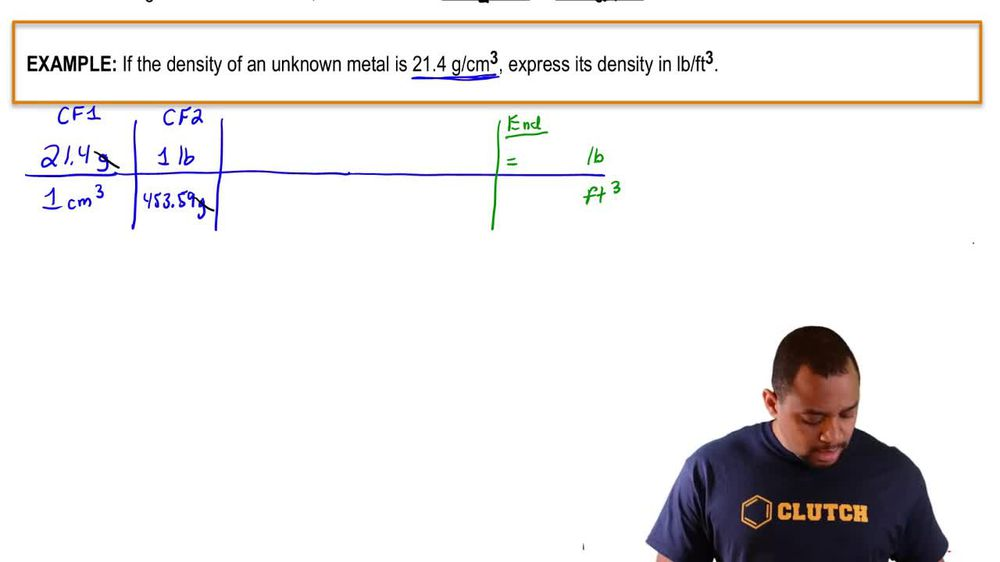Start typing, then use the up and down arrows to select an option from the list.
1. 1. Intro to General Chemistry2. Density
Problem

# Gold is alloyed (mixed) with other metals to increase its hardness in making jewelry. (b) The relative amount of gold in an alloy is commonly expressed in units of carats. Pure gold is 24 carat, and the percentage of gold in an alloy is given as a percentage of this value. For example, an alloy that is 50% gold is 12 carat. State the purity of the gold jewelry in carats.

Relevant Solution5m
Play a video:
Hi everyone here we have a question telling us that the amount of gold and an alloy is generally expressed in carrots. A bar of 24 karat gold is pure gold. 100%. A certain piece of gold jewelry contains only gold density equals 19.3 g per centimeter cube. And platinum density equals 21.4 g per centimeters cube. It has a mass of 11.4 g and a volume of 0.576 centimeters cubed. Our goal is to calculate the purity of the gold jewelry and carrots. So we're going to let X equal our massive gold And therefore 11.4 our total mass -X is going to give us our mass of platinum because our total volume equals our volume of gold plus our volume of platinum. Because that's all that is in this piece of jewelry. So zero .576 cm cubed is going to equal the mass of gold which we're going to denote as x grams times one centimeter cubed over 19.3 g plus 11.4 - times one centimeter cubed over Platinum's density 21. grams. So we need to multiply both sides by 19.3 and 21. to cancel it out. So 0.57, six Times 19. Times 21. equals x Times 21. plus 11. minus x Times 19.3. So that equals 237. Equals 21.4 x Plus 220. -19.3 x. So 237. 95, -220 .02 equals 2.1 x. So . equals 2.1 X. So divide both sides by 2.1, which gives us X equals 8. g, which gives us our mass of gold. So our percentage of gold Equals 8.51, Divided by 11.4 times 100 Which equals about 75%. So now we want to calculate our carrots, a whole carrot Or 100% gold is 24 Karats. So we have 24 carats And we want to multiply that by our percentage of gold, which is 75%, so times 75% equals 18 carats. So that is 18 Karat gold. And that is our final answer. Thank you for watching. Bye.